Updating search results...

# 43 Results

View
Selected filters:
• number-lineRating
0.0 stars

This website contains four practice problems dealing with absolute value. An answer key is provided.

Subject:
Mathematics
Material Type:
Activity/Lab
Lesson
Provider:
MathScore.com
Author:
MathScore.com
02/26/2019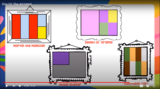Unrestricted Use
CC BY
Rating
0.0 stars

This lesson is Day 12 in a series of 12 lessons around fraction equivalences and comparisons.

Use your math skills to help detectives solve the crime. This video lesson reviews fractions of an area, fractions on a number line, and equivalent fractions. No materials are required.

Subject:
Mathematics
Material Type:
Activity/Lab
Interactive
Lesson
Simulation
Author:
Dawne Coker
06/25/2020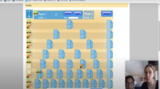Unrestricted Use
CC BY
Rating
0.0 stars

This lesson is Day 2 in a series of 12 lessons around fraction equivalences and comparisons.

This game may be played with one or two people. It reviews fractions on a number line, adding fraction with like denominators, and equivalent fractions. For an extra challenge, you can even break up your moves across multiple tracks (e.g., if your card reads 1/2, you could move 1/4 and 2/8).

https://www.nctm.org/Classroom-Resources/Illuminations/Interactives/Playing-Fraction-Track/

Subject:
Mathematics
Material Type:
Activity/Lab
Interactive
Lesson
Simulation
Author:
Dawne Coker
06/25/2020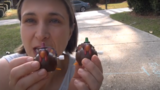Unrestricted Use
CC BY
Rating
0.0 stars

This lesson is Day 8 in a series of 12 lessons around fraction equivalences and comparisons.

This video lesson introduces the number as a tool for representing and comparing fractions. Students will need a black piece of a paper and a pencil with a good eraser.

Subject:
Mathematics
Material Type:
Activity/Lab
Interactive
Lesson
Simulation
Author:
Dawne Coker
06/25/2020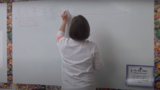Unrestricted Use
CC BY
Rating
0.0 stars

This lesson is Day 9 in a series of 12 lessons around fraction equivalences and comparisons.

This video lesson introduces students to fractions beyond 1 using a choral counting routine. Students connect the fractions to points on a number line and bar models. For this lesson, students need a piece of blank paper and a pencil.

Subject:
Mathematics
Material Type:
Activity/Lab
Interactive
Lesson
Simulation
Author:
Dawne Coker
06/25/2020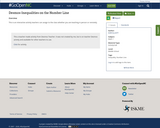Conditional Remix & Share Permitted
CC BY-NC
Rating
0.0 stars

This is an interactive activity&nbsp;teachers can assign to the class whether you are teaching in person or remotely.&nbsp;

Subject:
Math 1
Material Type:
Activity/Lab
Author:
KORI ELLIOTT
07/14/2020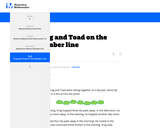Rating
0.0 stars

For this task, students use a number line to show why two different sums are equal .

Subject:
Mathematics
Material Type:
Activity/Lab
Provider:
Illustrative Mathematics
Author:
Illuminations Mathematics
02/26/2019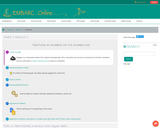Conditional Remix & Share Permitted
CC BY-NC-SA
Rating
0.0 stars

G3M5: Fractions as Numbers on the Number Line. Contains 30 Lessons.

Subject:
Mathematics
Material Type:
Module
Provider:
EMBARC.Online
08/13/2019Conditional Remix & Share Permitted
CC BY-NC
Rating
0.0 stars
Rating
0.0 stars
Subject:
Mathematics
Material Type:
Full Course
Provider:
Pearson
11/02/2020Conditional Remix & Share Permitted
CC BY-NC
Rating
0.0 stars

Equations and Inequalities

Type of Unit: Concept

Prior Knowledge

Students should be able to:

Add, subtract, multiply, and divide with whole numbers, fractions, and decimals.
Use the symbols &lt;, &gt;, and =.
Evaluate expressions for specific values of their variables.
Identify when two expressions are equivalent.
Simplify expressions using the distributive property and by combining like terms.
Use ratio and rate reasoning to solve real-world problems.
Order rational numbers.
Represent rational numbers on a number line.

Lesson Flow

In the exploratory lesson, students use a balance scale to find a counterfeit coin that weighs less than the genuine coins. Then continuing with a balance scale, students write mathematical equations and inequalities, identify numbers that are, or are not, solutions to an equation or an inequality, and learn how to use the addition and multiplication properties of equality to solve equations. Students then learn how to use equations to solve word problems, including word problems that can be solved by writing a proportion. Finally, students connect inequalities and their graphs to real-world situations.

Subject:
Algebra
Mathematics
Provider:
Pearson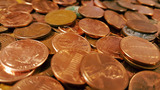Conditional Remix & Share Permitted
CC BY-NC
Rating
0.0 stars

Lesson OverviewStudents solve a classic puzzle about finding a counterfeit coin. The puzzle introduces students to the idea of a scale being balanced when the weight of the objects on both sides is the same and the scale being unbalanced when the objects on one side do not weigh the same as the objects on the other side.Key ConceptsThe concept of an inequality statement can be modeled using an unbalanced scale. The context—weighing a set of coins in order to identify the one coin that weighs less than the others—allows students to manipulate the weight on either side of the scale. In doing so, they are focused on the relationship between two weights—two quantities—and whether or not they are equal.Goals and Learning ObjectivesExplore a balance scale as a model for an equation or an inequality.Introduce formal meanings of equality and inequality.

Material Type:
Lesson Plan
Author:
Pearson
11/02/2020Conditional Remix & Share Permitted
CC BY-NC
Rating
0.0 stars

Lesson OverviewStudents represent inequalities on a number line, find at least one value that makes the inequality true, and write the inequality using words.SWD:When calling on students, be sure to call on ELLs and to encourage them to actively participate. Understand that their pace might be slower or they might be shy or more reluctant to volunteer due to their weaker command of the language.SWD:Thinking aloud is one strategy for making learning visible. When teachers think aloud, they are externalizing their internal thought processes. Doing so may provide students with insights into mathematical thinking and ways of tackling problems. It also helps to model accurate mathematical language.Key ConceptsInequalities, like equations, have solutions. An arrow on the number line—pointing to the right for greater values and to the left for lesser values—can be used to show that there are infinitely many solutions to an inequality.The solutions to x &lt; a are represented on the number line by an arrow pointing to the left from an open circle at a.Example: x &lt; 2The solutions to x &gt; a are represented on the number line with an arrow pointing to the right from an open circle at a.Example: x &gt; 2The solutions to x ≤ a are represented on the number line with an arrow pointing to the left from a closed circle at a.Example: x ≤ 2The solutions to x ≥ a are represented on the number line with an arrow pointing to the right from a closed circle at a.Example: x ≥ 2Goals and Learning ObjectivesRepresent an inequality on a number line and using words.Understand that inequalities have infinitely many solutions.

Material Type:
Lesson Plan
Author:
Pearson
11/02/2020Conditional Remix & Share Permitted
CC BY-NC
Rating
0.0 stars

Getting Started

Type of Unit: Introduction

Prior Knowledge

Students should be able to:

Solve and write numerical equations for whole number addition, subtraction, multiplication, and division problems.
Use parentheses to evaluate numerical expressions.
Identify and use the properties of operations.

Lesson Flow

In this unit, students are introduced to the rituals and routines that build a successful classroom math community and they are introduced to the basic features of the digital course that they will use throughout the year.

An introductory card sort activity matches students with their partner for the week. Then over the course of the week, students learn about the lesson routines: Opening, Work Time, Ways of Thinking, Apply the Learning, Summary of the Math, and Reflection. Students learn how to present their work to the class, the importance of taking responsibility for their own learning, and how to effectively participate in the classroom math community.

Students then work on Gallery problems to further explore the program’s technology resources and tools and learn how to organize their work.

The mathematical work of the unit focuses on numerical expressions, including card sort activities in which students identify equivalent expressions and match an expression card to a word card that describes its meaning. Students use the properties of operations to identify equivalent expressions and to find unknown values in equations.

Subject:
Mathematics
Provider:
PearsonConditional Remix & Share Permitted
CC BY-NC
Rating
0.0 stars

Gallery OverviewAllow students who have a clear understanding of the content thus far in the unit to work on Gallery problems of their choosing. You can then use this time to provide additional help to students who need review of the unit's concepts or to assist students who may have fallen behind on work.Gallery DescriptionRepresent a Math ProblemStudents explore the number line tool and the double number line tool. They use the number line tool to solve a problem about the weights of a cheetah and a fisher cat.Research ExpressionsStudents learn the difference between numerical expressions and variable expressions. They watch video tutorials, review worked examples, use the Glossary, and explore other resources.Fish TankStudents create diagrams and use text and images as they solve a problem about the size of a fish tank.

Material Type:
Lesson Plan
Author:
Pearson
11/02/2020Conditional Remix & Share Permitted
CC BY-NC
Rating
0.0 stars

Rational Numbers

Type of Unit: Concept

Prior Knowledge

Students should be able to:

Solve problems with positive rational numbers.
Plot positive rational numbers on a number line.
Understand the equal sign.
Use the greater than and less than symbols with positive numbers (not variables) and understand their relative positions on a number line.
Recognize the first quadrant of the coordinate plane.

Lesson Flow

The first part of this unit builds on the prerequisite skills needed to develop the concept of negative numbers, the opposites of numbers, and absolute value. The unit starts with a real-world application that uses negative numbers so that students understand the need for them. The unit then introduces the idea of the opposite of a number and its absolute value and compares the difference in the definitions. The number line and positions of numbers on the number line is at the heart of the unit, including comparing positions with less than or greater than symbols.

The second part of the unit deals with the coordinate plane and extends student knowledge to all four quadrants. Students graph geometric figures on the coordinate plane and do initial calculations of distances that are a straight line. Students conclude the unit by investigating the reflections of figures across the x- and y-axes on the coordinate plane.

Subject:
Mathematics
Provider:
PearsonConditional Remix & Share Permitted
CC BY-NC
Rating
0.0 stars

Students watch a video showing the highest and lowest locations on each of the continents. Then they create a diagram (a number line) for a book titled The World’s Highest and Lowest Locations. Students show four of the highest elevations and four of the lowest elevations in the world on their diagrams.Key ConceptsA complete number line has both positive numbers (to the right of 0) and negative numbers (to the left of 0).Negative numbers are written with a minus sign—for example, –12, which is pronounced “negative 12.”Positive numbers can be written with a plus sign for emphasis, such as +12, but a number without a sign, such as 12, is always interpreted as positive.Every number except 0 is either positive or negative. The number 0 is neither positive nor negative.Goals and Learning ObjectivesCreate a number line to show elevations that are both above and below sea level.

Material Type:
Lesson Plan
Author:
Pearson
11/02/2020Conditional Remix & Share Permitted
CC BY-NC
Rating
0.0 stars

Students watch a dot get tossed from one number on a number line to the opposite of the number. Students predict where the dot will land each time based on its starting location.Key ConceptsThe opposite of a number is the same distance from 0 as the number itself, but on the other side of 0 on a number line.In the diagram, m is the opposite of n, and n is the opposite of m. The distance from m to 0 is d, and the distance from n to 0 is d; this distance to 0 is the same for both n and m. The absolute value of a number is its distance from 0 on a number line.Positive numbers are numbers that are greater than 0.Negative numbers are numbers that are less than 0.The opposite of a positive number is negative, and the opposite of a negative number is positive.Since the opposite of 0 is 0 (which is neither positive nor negative), then 0 = 0. The number 0 is the only number that is its own opposite.Whole numbers and the opposites of those numbers are all integers.Rational numbers are numbers that can be expressed as ab, where a and b are integers and b ≠ 0.Goals and Learning ObjectivesIdentify a number and its oppositeLocate the opposite of a number on a number lineDefine the opposite of a number, negative numbers, rational numbers, and integers

Material Type:
Lesson Plan
Author:
Pearson
11/02/2020Conditional Remix & Share Permitted
CC BY-NC
Rating
0.0 stars

Four full-year digital course, built from the ground up and fully-aligned to the Common Core State Standards, for 7th grade Mathematics. Created using research-based approaches to teaching and learning, the Open Access Common Core Course for Mathematics&nbsp;is designed with student-centered learning in mind, including activities for students to develop valuable 21st century skills and academic mindset.

Subject:
Mathematics
Material Type:
Full Course
Provider:
Pearson
11/02/2020Conditional Remix & Share Permitted
CC BY-NC
Rating
0.0 stars

Working With Rational Numbers

Type of Unit: Concept

Prior Knowledge

Students should be able to:

Compare and order positive and negative numbers and place them on a number line.
Understand the concepts of opposites absolute value.

Lesson Flow

The unit begins with students using a balloon model to informally explore adding and subtracting integers. With the model, adding or removing heat represents adding or subtracting positive integers, and adding or removing weight represents adding or subtracting negative integers.

Students then move from the balloon model to a number line model for adding and subtracting integers, eventually extending the addition and subtraction rules from integers to all rational numbers. Number lines and multiplication patterns are used to find products of rational numbers. The relationship between multiplication and division is used to understand how to divide rational numbers. Properties of addition are briefly reviewed, then used to prove rules for addition, subtraction, multiplication, and division.

This unit includes problems with real-world contexts, formative assessment lessons, and Gallery problems.

Subject:
Algebra
Mathematics
Provider:
PearsonConditional Remix & Share Permitted
CC BY-NC
Rating
0.0 stars